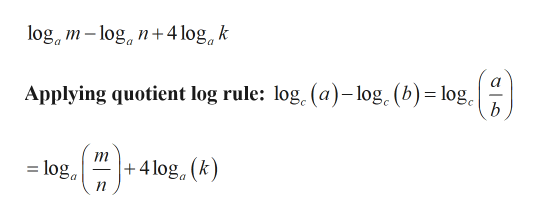# Use the product, quotient, and power rules of logarithms to rewrite the expression as a single logarithm, assume that all variables represent positive real numbers:log a^m - log a^n + 4 log a^kThank you

Question
30 views

Use the product, quotient, and power rules of logarithms to rewrite the expression as a single logarithm, assume that all variables represent positive real numbers:

log a^m - log a^n + 4 log a^k

Thank you

check_circle

Step 1

Given:

Step 2

Calculation:

We have to convert into single logarithm.help_outlineImage Transcriptioncloselog m-log n4 log k a Applying quotient log rule: log, (a)-log. (b) log b 4 lg, (k) =loga + fullscreen
Step 3

### Want to see the full answer?

See Solution

#### Want to see this answer and more?

Solutions are written by subject experts who are available 24/7. Questions are typically answered within 1 hour.*

See Solution
*Response times may vary by subject and question.
Tagged in

### Logarithms